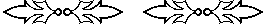## The Egyptian 2/n table, the recto table of the Ahmes (Rhind) papyrus

The Egyptian concept of fraction requires that any fraction be represented as a sum of unit fractions without any repetitions, except 2/3 which was allowed. Thus, for example, our common fraction 2/5 would be treated as a problem, not as an answer. The problem is to divide 2 by 5; the answer would be any sum of unit fractions without repetition. One answer is 1/3 + 1/15, the preferred answer. Another possible answer would be 1/4 + 1/10 + 1/20, but that's a more complicated answer having both more terms and larger denominators. Note that 1/5 + 1/5 would not be an answer because 1/5 is repeated.

The Egyptian algorithms for mulitplication and division are based on addition, subtraction, and doubling. Therefore, one ingrediant necessary to compute products and quotients involving fractions is a table of doubles of unit fractions. It's also necessary for addition since when adding two sums of unit fractions, some particular unit fraction might occur twice.

The back (recto) of the most important Egyptian mathematical papyrus, the Ahmes, or Rhind, papyrus, includes a table of doubles of unit fractions. We can call it a 2/n table. Here it is, transcribed into modern numerals. Note that only the denominators are listed in this transcription. In one column appears the denominator of the unit fraction to be doubled, and in the next column appear the denominators of the unit fractions for that double.

 5 3 15 7 4 28 9 6 18 11 6 66 13 8 52 104 15 10 30 17 12 51 68 19 12 76 114 21 14 42 23 12 276 25 15 75 27 18 54 29 24 58 174 232 31 20 124 155 33 22 66 35 30 42 37 24 111 296
 39 26 78 41 24 246 328 43 42 86 129 301 45 30 60 47 30 141 470 49 28 196 51 34 102 53 30 318 795 55 30 330 57 38 114 59 36 236 531 61 40 244 488 610 63 42 126 65 39 195 67 40 355 536 69 46 138
 71 40 568 710 73 60 219 292 365 75 50 150 77 44 308 79 60 237 316 790 81 54 162 83 60 332 415 498 85 51 255 87 58 174 89 60 356 534 890 91 70 130 93 62 186 95 60 380 570 97 56 679 776 99 66 198 101 101 202 303 606

At first glance, the only apparent regularity in the table occurs for denominators divisible by 3, and for those the rule is:

 23n = 12n + 16n

Upon further analysis, you can perceive other principles used in constructing the table.